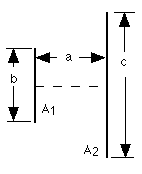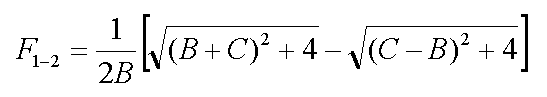Cody

# Problem 2806. Radiation Heat Transfer — View Factors (2)

Created by goc3 in Community

View factors (aka configuration factors) are utilized in some radiation heat transfer models to estimate heat transfer rates between surfaces. In particular, the thermal energy leaving a given surface is applied to other surfaces, as appropriate, based on how much the hot surface "sees" the other surfaces. As such, view factors are purely geometrical in nature. A range of view factor formulae are available here.

For this problem, calculate the view factor from surface 1 to surface 2 (F_1-2) for two directly opposed, infinitely long plates of different finite widths given the distance between the plates:Each variable may be a vector of values. Also, note that B = b/a and C = c/a.

### Solution Stats

34.25% Correct | 65.75% Incorrect
Last solution submitted on Jan 24, 2019